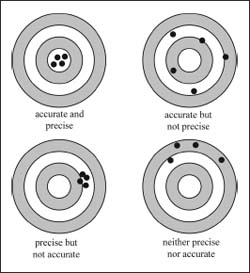NextPrevious

# What are some common units of concentration for a species in solution?

The most common unit of concentration in solution-phase chemistry is molarity, or the number of moles of a species present per liter of solution. Recall that the number of moles of a species is equal to the number of molecules of that species divided by Avogadro’s number (see “History of Chemistry” for information on Avogadro’s number).In analytical chemistry, it can often be more convenient to discuss concentrations in terms of other units. This is particularly true when the species being studied is present in very small concentrations. Some other commonly used units are parts per million (ppm), parts per billion, or parts per trillion. These units refer to the mass fraction of the analyte in question to total mass of the sample. One part per million means 1 millionth (10−6) of a gram of the species in question, or analyte, per gram of the sample present. Similarly, one part per billion means 1 billionth (10−9) of a gram of analyte per gram of sample, and one part per trillion means 1 trillionth (10−12) of a gram of analyte per gram of sample. It is also possible to use each of these definitions based on a per unit volume basis, rather than a per unit mass basis (though this is more common in the gas phase). For example, 1 ppm by volume of an analyte means the analyte occupies 1 millionth (10−6) of the total volume of a sample.A meniscus is the curve that forms on the surface of a liquid in a container. It can make measuring liquids by eye somewhat of a challenge.

Close

This is a web preview of the "The Handy Chemistry Answer Book" app. Many features only work on your mobile device. If you like what you see, we hope you will consider buying. Get the App